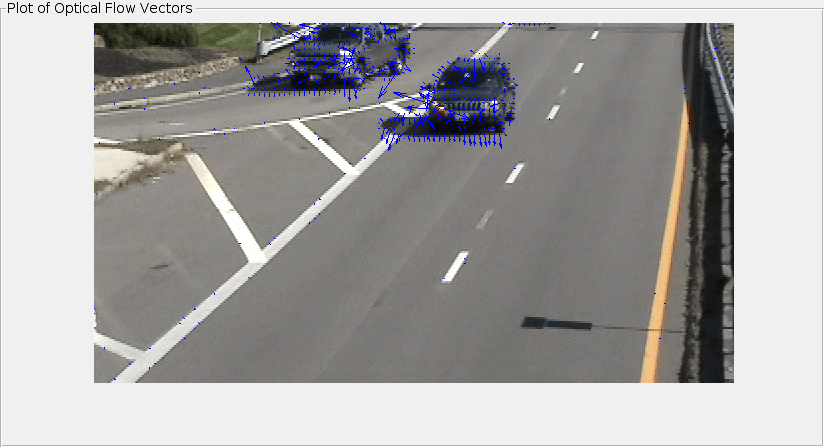Documentation

opticalFlowHS

Object for estimating optical flow using Horn-Schunck method

Description

Create an optical flow object for estimating the direction and speed of a moving object using the Horn-Schunck method. Use the object function estimateFlow to estimate the optical flow vectors. Using the reset object function, you can reset the internal state of the optical flow object.

Creation

Description

opticFlow = opticalFlowHS returns an optical flow object that you can use to estimate the direction and speed of the moving objects in a video. The optical flow is estimated using the Horn-Schunck method.

example

opticFlow = opticalFlowHS(Name,Value) returns an optical flow object with properties specified as one or more Name,Value pair arguments. Any unspecified properties have default values. Enclose each property name in quotes.

For example, opticalFlowHS('Smoothness',1.5)

Properties

expand all

Expected smoothness of optical flow, specified as a positive scalar. Increase this value when there is increased motion between consecutive frames. A typical value for 'Smoothness' is around 1.

Maximum number of iterations, specified as a positive integer-valued scalar. Increase this value to estimate the optical flow of objects with low velocity.

The iterative computation stops when the number of iterations equals the value of 'MaxIteration' or when the algorithm reaches the value set for 'VelocityDifference'. To stop computation only by using 'MaxIteration', set the value of 'VelocityDifference' to 0.

Minimum absolute velocity difference, specified as a positive scalar. This value depends on the input data type. Decrease this value to estimate the optical flow of objects that have low velocity.

The iterative computation stops when the algorithm reaches the value set for 'VelocityDifference' or the number of iterations equals 'MaxIteration'. To use only 'VelocityDifference' to stop computation, set 'MaxIteration' to Inf.

Object Functions

 estimateFlow Estimate optical flow reset Reset the internal state of the optical flow estimation object

Examples

collapse all

Create a VideoReader object for the input video file, visiontraffic.avi. Specify the timestamp of the frame to read as 11.

Specify the optical flow estimation method as opticalFlowHS. The output is an object specifying the optical flow estimation method and its properties.

opticFlow = opticalFlowHS
opticFlow =
opticalFlowHS with properties:

Smoothness: 1
MaxIteration: 10
VelocityDifference: 0

Create a custom figure window to visualize the optical flow vectors.

h = figure;
movegui(h);
hViewPanel = uipanel(h,'Position',[0 0 1 1],'Title','Plot of Optical Flow Vectors');
hPlot = axes(hViewPanel);

Read image frames from the VideoReader object and convert to grayscale images. Estimate the optical flow from consecutive image frames. Display the current image frame and plot the optical flow vectors as quiver plot.

frameGray = rgb2gray(frameRGB);
flow = estimateFlow(opticFlow,frameGray);
imshow(frameRGB)
hold on
plot(flow,'DecimationFactor',[5 5],'ScaleFactor',60,'Parent',hPlot);
hold off
pause(10^-3)
endAlgorithms

expand all

To compute the optical flow between two images, you must solve this optical flow constraint equation:

${I}_{x}u+{I}_{y}v+{I}_{t}=0$

.

• ${I}_{x}$, ${I}_{y}$, and ${I}_{t}$ are the spatiotemporal image brightness derivatives.

• u is the horizontal optical flow.

• v is the vertical optical flow.

 Barron, J. L., D. J. Fleet, S. S. Beauchemin, and T. A. Burkitt. “ Performance of optical flow techniques.” In Proceedings of the IEEE Conference on Computer Vision and Pattern Recognition (CVPR),236-242. Champaign, IL: CVPR, 1992.